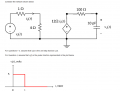# Voltage in an RC Circuit

#### libnitz

Joined Jan 29, 2016
17
So I had this question on a quiz that I bombed and I'm still confused over it.What is the steady-state value of the capacitor voltage v(infinity) when excited by the unit step function source?

The way I went around the problem was to find ib(t) which is ib(t) = 5/(4 +1) = 1 A.

I then used the ib(t) to find the voltage source which would be the voltage at infinity (with the capacitor replaced by an open circuit)
Therefore the voltage should be V = 12ib(t) = 12 (1) = 12 V.

I was wrong and I'm not sure why.

Thanks.

PS: Was I wrong in assuming the Rth would be 100 ohms since the left half of the circuit would not contribute?

#### shteii01

Joined Feb 19, 2010
4,644
If the cap is fully charged and modeled as an open circuit, like you did, then the dependent current source is not part of a complete circuit, then there is no current.

If I am right, then the Vo(t) is voltage across the 4 Ohm resistor, which is Vo(t)=4*5/(4+1)=4 volt.

#### libnitz

Joined Jan 29, 2016
17
If the cap is fully charged and modeled as an open circuit, like you did, then the dependent current source is not part of a complete circuit, then there is no current.

If I am right, then the Vo(t) is voltage across the 4 Ohm resistor, which is Vo(t)=4*5/(4+1)=4 volt.
Hmm that's sorta threw me in a loop; Regardless, the options were a. 2.4V b. 1 V c. 9.6 V and d. 0V

I remember picking 9.6 V but can't remember why.

#### shteii01

Joined Feb 19, 2010
4,644
Hmm that's sorta threw me in a loop; Regardless, the options were a. 2.4V b. 1 V c. 9.6 V and d. 0V

I remember picking 9.6 V but can't remember why.
Ok, so you picked 9.6 volt first time and it was wrong, I did 4 volt but it is not even a choice, you also did 12 volt and it is also not a choice.

I think it leaves only 0 volt. I am not sure I have a good explanation.

#### RBR1317

Joined Nov 13, 2010
706
Isn't the magnitude of the UNIT step function equal to 1?

That would make Ib=0.2 and Vo= 0.2*12 = 2.4 V.

#### libnitz

Joined Jan 29, 2016
17
Isn't the magnitude of the UNIT step function equal to 1?

That would make Ib=0.2 and Vo= 0.2*12 = 2.4 V.
Could you please explain how you got your current ib as 0.2? Did you get this information from the graph?

#### RBR1317

Joined Nov 13, 2010
706

#### shteii01

Joined Feb 19, 2010
4,644
Isn't the magnitude of the UNIT step function equal to 1?

That would make Ib=0.2 and Vo= 0.2*12 = 2.4 V.
Yes, that makes sense. I need to double check the definition of unit step.

#### WBahn

Joined Mar 31, 2012
28,182
The problem says that the voltage is a unit step for questions 1-3 and a 5 V pulse for question 4. But you never give any hint regarding which question it is you are trying to answer. But if you are using a unit step, then you need to consider what the magnitude is of a "unit" step function.

•libnitz